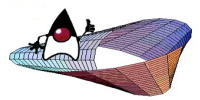numEclipse - An Eclipse based workbench for

Numerical Computing

# Differential Equations

This chapter presents two functions for solving ordinary differential equations (ODEs).

## Ordinary Differential Equations

numEclipse implements two methods for solving ODE, i.e., ode45 and ode78. These open source functions are adopted from GNU Octave.

[T,Y] = ode45(func,ts,x0)

[T,Y] = ode78(func,ts,x0)

Both of these functions solve initial value problems for ODEs. ode45 uses 4rth and 5th order Runga-Kutta methods, whereas ode78 uses order 7 and 8 methods.

Here is an example of how to use ode45 function.

#func.m

# This function evaluates the population of competing species.

function y = func(t, x)
u = x(1);
v = x(2);
y = zeros(2, 1);
y(1) = 3 * u - (5/2) * u * v;
y(2) = 2 * v -  u * v;

> ts = [0 2.5];

> x0 = [0.2 0.18];

> [t, y] = ode45(@func, ts, x0);

> plot(t, y(:, 1))

Following plot shows the population evolution for specie 1.> plot(t, y(:, 2))

Following plot shows the population evolution for specie 2.